SCERT AP 7th Class Maths Solutions Pdf Chapter 6 Data Handling Ex 6.3 Textbook Exercise Questions and Answers.

## AP State Syllabus 7th Class Maths Solutions 6th Lesson Data Handling Ex 6.3

Question 1.
Find the Median of the following:
(i) 7, 3, 15, 0, 1, 71, 19, 4, 17.
Given data : 7, 3, 15. 0, 1, 71, 19, 4, 17.
Arrange the given observations in ascending order.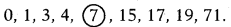In nine observations the fifth observation 7 is the middle most value.
∴ Median = 7(ii) 12, 23, 11, 18, 15, 20, 86, 27.
Given data : 12, 23, 11, 18, 15, 20, 86, 27.
Arrange the given observations in ascending order: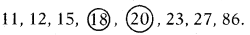In eight observations the 4th and 5th observations are 18 and 20.
Here, we have two middle most values 18 and 20.
Median = Average of the two middle most values.
= $$\frac{18+20}{2}$$ = $$\frac{38}{2}$$ = 19
∴ Median of the data = 19.

Question 2.
The number of pages in text books of different subjects are 421, 175, 128, 117, 150, 145, 147 and 113 find median of given data.
Given data : 421, 175, 128, 117, 150, 145, 147, 113
Arrange the given observations in ascending order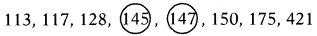In eight observations the 4th and 5th observations are 145 and 147.
Here, we have two middle most values 145 and 147.
Median = Average of the two middle most values
= $$\frac{145+147}{2}$$ = $$\frac{292}{2}$$ = 146
∴ Median of the data = 146.

Question 3.
The weekly sales of motor bikes in a showroom for the past 14 weeks are 10, 6, 8, 3, 5, 6, 4, 7, 12, 13, 16, 10, 4 and 7. Find the Median of the data.
Given data: 10, 6, 8, 3, 5, 6, 4, 7, 12, 13, 16, 10, 4, 7
Arrange the given observations in ascending order.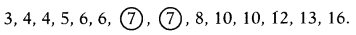In fourteen observations the 7th and 8th observations are 7 and 7.
Here we have two middle most values 7 and 7.
Median = Average of the two middle most values
= $$\frac{7+7}{2}$$ = $$\frac{14}{2}$$ = 7
∴ Median of the data = 7Question 4.
Find the Median of 0.3, 0.25, 0.32, 0.147, 0.19, 0.2 and 7.1.
Given data: 0.3, 0.25, 0.32, 0.147, 0.19, 0.2, 7.1.
Arrange the given observations in ascending order.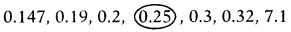In seven observations the 4th observation 0.25 is the middle most value.
∴ Median. = 0.25

Question 5.
If the Median of observations 2x, 3x, 4x, 5x, 6x, (x > 0) is 28, then find the value of ‘x’.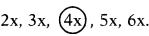⇒ $$\frac{4 x}{4}$$ = $$\frac{28}{4}$$ = 7